• 图像分类任务
• 收集训练集与测试集
• 支持向量机分类基本原理
• 特征选择与提取
• SVM 进行图像分类
• 分类结果评价

# 1. 图像分类任务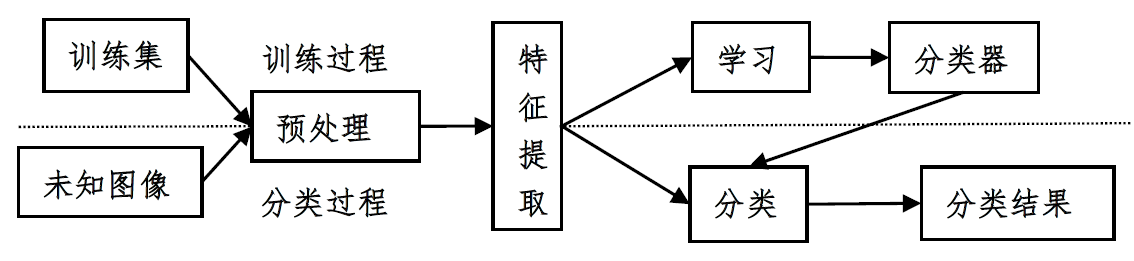# 2. 收集训练集与测试集

cifar10图像集是常用的图像分类数据集，由多伦多大学的Geoffrey Hinton教授等人收集整理，包含了60000张大小为32*32的彩色图像，其中50000张作为训练数据，10000张用于测试数据。50000张训练图片分为5个子集，分别命名为data_batch_1~5。每个子集都包含正好10000张图片，cifar10包含的图片类别如下图所示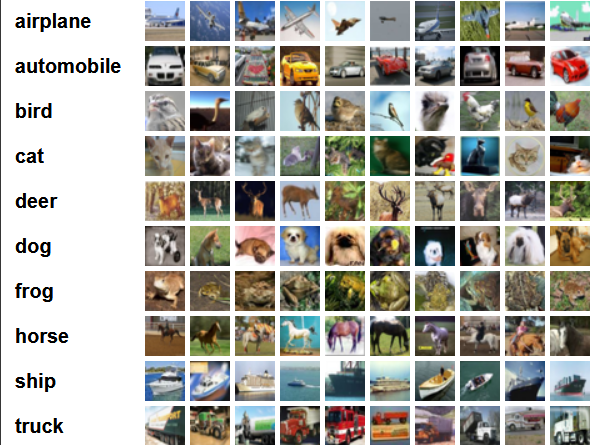# 3. 支持向量机分类基本原理

SVM是以最优化理论为基础来处理机器学习的新方式。它的提出主要是用来解决两类分类的问题，在两类中寻找一个最优超分平面将两类分开，来提高分类的正确率。

wxb=0  w ⋅ x − b = 0
<script type="math/tex; mode=display" id="MathJax-Element-3"> w\cdot x-b=0\ </script> 对其进行归一化，使得样本
(xi,yi),i=1,...,n,xϵRd,yϵ{+1,1} ( x i , y i ) , i = 1 , . . . , n , x ϵ R d , y ϵ { + 1 , − 1 }
<script type="math/tex; mode=display" id="MathJax-Element-4">(x_{i},y_{i}),i=1,...,n,x\epsilon R^{d},y\epsilon \left \{ +1,-1 \right \}</script>满足
yi[(w,xi)b]10,i=1,...,n y i [ ( w , x i ) − b ] − 1 ≥ 0 , i = 1 , . . . , n
<script type="math/tex; mode=display" id="MathJax-Element-5">y_{i}[(w,x_{i})-b]-1\geq 0,i=1,...,n</script>是表示的是最优分类超平面，图中m1为分类超平面，m2、m3分别为与超分平面平行且是过两类中离分类超平面最近的样本，他们间的距离称作分类间隔，间距为 2/w 2 / ‖ w ‖ <script type="math/tex" id="MathJax-Element-6">2/‖w‖</script>。

## 3.1 线性可分情况

min:J(w,b,a)=12wTwi=1Nai[yi(wTxi+b)1] m i n : J ( w , b , a ) = 1 2 w T w − ∑ i = 1 N a i [ y i ( w T x i + b ) − 1 ]
<script type="math/tex; mode=display" id="MathJax-Element-12">min :J(w,b,a)=\frac{1}{2}w^{T}w-\sum_{i=1}^{N} a_{i}[y_{i}(w^{T}x_{i}+b)-1]</script>
yi(ωTixi+b)+1 y i ( ω i T x i + b ) ≥ + 1
<script type="math/tex; mode=display" id="MathJax-Element-13">y_i (ω_i^T x_i+b)≥+1</script>

Q(a)=j=1Nai12i=1Nj=1Naiajyiyj(xi,xj) Q ( a ) = ∑ j = 1 N a i − 1 2 ∑ i = 1 N ∑ j = 1 N a i a j y i y j ( x i , x j )
<script type="math/tex; mode=display" id="MathJax-Element-15">Q(a)=\sum_{j=1}^{N}a_{i}-\frac{1}{2} \sum_{i=1}^{N} \sum_{j=1}^{N} a_{i} a_j y_i y_j (x_i,x_j )</script>此式称为式(1)的对偶形式。同时，优化问题的最优解必须满足如下KKT条件： aiyi(ωTxi+b)1=0 a i y i ( ω T x i + b ) − 1 = 0 <script type="math/tex" id="MathJax-Element-16">a_i {y_i (ω^T x_i+b)-1}=0</script>，任意 ai0 a i ≠ 0 <script type="math/tex" id="MathJax-Element-17">a_i≠0</script>，可求得b。因为 Ni=1aiyi=0 ∑ i = 1 N a i y i = 0 <script type="math/tex" id="MathJax-Element-18">\sum_{i=1}^{N}a_i y_i=0 </script>，结果可得大部分 ai a i <script type="math/tex" id="MathJax-Element-19">a_i</script>等于0，将 ai a i <script type="math/tex" id="MathJax-Element-20">a_i</script>不等于0所对应的样本称为支持向量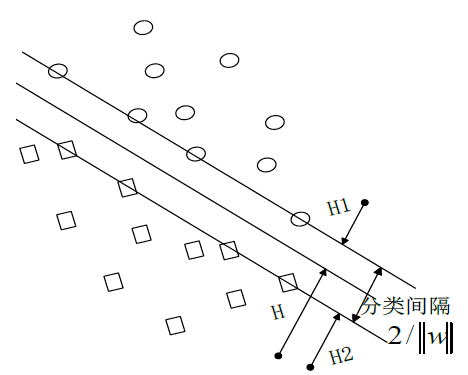## 3.2 非线性可分

max:Q(a)=j=1Nai12i=1Nj=1Naiajyiyj(xi,xj) m a x : Q ( a ) = ∑ j = 1 N a i − 1 2 ∑ i = 1 N ∑ j = 1 N a i a j y i y j ( x i , x j )
<script type="math/tex; mode=display" id="MathJax-Element-22">max:Q(a)=\sum_{j=1}^{N}a_{i}-\frac{1}{2} \sum_{i=1}^{N} \sum_{j=1}^{N} a_{i} a_j y_i y_j (x_i,x_j )</script>
i=1Naiyi=0,ai0,i=1,2,...,N ∑ i = 1 N a i y i = 0 , a i ≥ 0 , i = 1 , 2 , . . . , N
<script type="math/tex; mode=display" id="MathJax-Element-23">\sum_{i=1}^{N}a_i y_i=0,a_i≥0,i=1,2,...,N</script>其中， [φ(xi),φ(xj)] [ φ ( x i ) , φ ( x j ) ] <script type="math/tex" id="MathJax-Element-24">[φ(x_i ),φ(x_j)]</script>是]是变换后的空间内积。此时，我们并不知道 φ(x) φ ( x ) <script type="math/tex" id="MathJax-Element-25">φ(x)</script>的具体形式。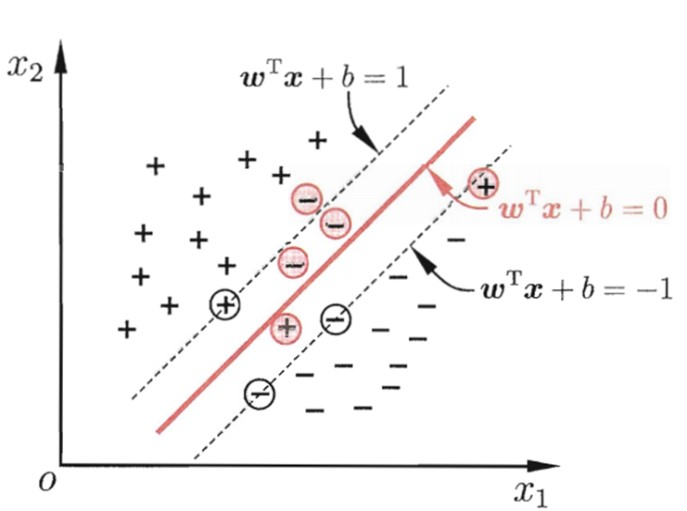• 多项式核函数： k(x,xi)=[(xxi)+1]q k ( x , x i ) = [ ( x ⋅ x i ) + 1 ] q <script type="math/tex" id="MathJax-Element-30">k(x,x_i )=[(x\cdot x_i )+1]^q</script>；
• 基于径向基函数RBF核函数形式： k(x,xi)=exp|xxi|2/σ2 k ( x , x i ) = e x p ⁡ − | x − x i | 2 / σ 2 <script type="math/tex" id="MathJax-Element-31">k(x,x_i )=exp⁡{-|x-x_i |^2/σ^2}</script>；
• Sigmoid核函数： k(x,xi)=tanh[v(xxi)+c] k ( x , x i ) = t a n h ⁡ [ v ( x ⋅ x i ) + c ] <script type="math/tex" id="MathJax-Element-32">k(x,x_i )=tanh⁡[v(x\cdot x_i )+c]</script>。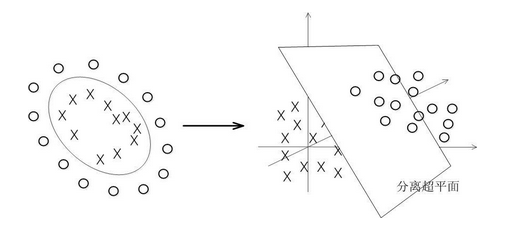SVM数据集形成的分类函数具有这样的性质：它是一组以支持向量为参数的非线性函数的线性组合。因此，分类函数的表达式仅和支持向量的数量有关，而独立于空间的维度。在处理高维输入空间的分类时，这种方法尤其有效。

## 3.3 一对一SVM(1-VS-1 SVMS)

fst(x)=wstϕ(x)+bst=i=svastiyik(xi,x)+bst f s t ( x ) = w s t ⋅ ϕ ( x ) + b s t = ∑ i = s v a i s t y i k ( x i , x ) + b s t
<script type="math/tex; mode=display" id="MathJax-Element-34">f_{st} (x)=w_{st}\cdot \phi (x)+b_st =\sum_{i=sv}a_{i}^{st}y_{i}k(x_{i},x)+b_{st}</script>这种方式的优点：对于每个子 SVM S V M <script type="math/tex" id="MathJax-Element-35">SVM</script>，由于训练样本少，因此其训练速度显而易见快于l对多SVM方式，同时其精度也较高。这种方式的缺点：随着类数K的增多，SVM的个数也越来越大，随着k个数的增多，其训练速度也会越来越慢，这是需要改进的地方。

# 4. 特征选择与提取

## 4.1 方向梯度直方图

HOG特征描述子，它通过计算和统计图像局部区域的梯度方向直方图来构成特征，广泛应用于图像处理中进行物体检测。提取HOG特征包括以下几个步骤：

1) 归一化图像。首先把输入的彩色图像转灰度图像，然后对图像进行平方根Gamma压缩，从而达到归一化效果。这种压缩处理能够有效地降低图像局部的阴影和光照变化，从而提高HOG特征对于光照变化的鲁棒性。

2) 计算图像梯度。首先用一维离散微分模版[-1,0,1]及其转置分别对归一化后的图像进行卷积运算，得到水平方向的梯度分量以及垂直方向的梯度分量。然后根据当前像素点的水平梯度和垂直梯度，得到当前像素点的梯度幅值和梯度方向。公式如下：

Gx(x,y)=H(x+1,y)H(x1,y) G x ( x , y ) = H ( x + 1 , y ) − H ( x − 1 , y )
<script type="math/tex; mode=display" id="MathJax-Element-36">G_x (x,y)=H(x+1,y)-H(x-1,y)</script>
Gy(x,y)=H(x,y+1)H(x,y1) G y ( x , y ) = H ( x , y + 1 ) − H ( x , y − 1 )
<script type="math/tex; mode=display" id="MathJax-Element-37">G_y (x,y)=H(x,y+1)-H(x,y-1)</script>
G(x,y)=Gx(x,y)2+Gy(x,y)2 G ( x , y ) = G x ( x , y ) 2 + G y ( x , y ) 2
<script type="math/tex; mode=display" id="MathJax-Element-38">G(x,y)=\sqrt{G_x (x,y)^2+G_y (x,y)^2}</script>
α(x,y)=tan1((Gy(x,y))/(Gx(x,y))) α ( x , y ) = t a n − 1 ( ( G y ( x , y ) ) / ( G x ( x , y ) ) )
<script type="math/tex; mode=display" id="MathJax-Element-39">α(x,y)=tan^{-1} ((G_y (x,y))/(G_x (x,y)))</script>其中， Gx(x,y)Gy(x,y)H(x,y) G x ( x , y ) ， G y ( x , y ) ， H ( x , y ) <script type="math/tex" id="MathJax-Element-40">G_x (x,y)，G_y (x,y)，H(x,y)</script>分别表示当前像素点的水平梯度，垂直梯度和像素值。 G(x,y)α(x,y) G ( x , y ) ， α ( x , y ) <script type="math/tex" id="MathJax-Element-41">G(x,y)，α(x,y)</script>分别为当前像素点的梯度幅值和梯度方向。

3) 为每个细胞单元构建梯度方向直方图。首先把尺寸为64×128的图像分为8×16个cell，即是每个cell为8×8个像素。然后把梯度方向限定在[0, π]，并将梯度方向平均分为9个区间（bin），每个区间20度。最后对cell内每个像素用梯度方向在直方图中进行加权投影，也就是说cell中的每个像素点都根据该像素点的梯度幅值为某个方向的bin进行投票，这样就可以得到这个cell的梯度方向直方图，也就是该cell对应的9维特征向量。

4) 把细胞单元组合成大的块（block），块内归一化梯度直方图。把相邻的2×2个cell形成一个block，这样每个block就对应着36维的特征向量。由于局部光照的变化以及前景和背景对比度的变化，使得梯度强度的变化范围非常大。为了进一步消除光照的影响，最后对block内的36维特征向量进行归一化。公式如下：

V=vv22+ε2 V = v ‖ v ‖ 2 2 + ε 2
<script type="math/tex; mode=display" id="MathJax-Element-42"> V=\frac{v}{\sqrt{\left \| v \right \|_2^2+\varepsilon ^{2}}}</script>其中，v 是未经归一化的描述子向量 v2 ‖ v ‖ 2 <script type="math/tex" id="MathJax-Element-43">‖v‖_2</script>是v的2范数，ε是一个极小的常数。

5) 收集HOG特征。如图1所示，采用滑动窗口的方法，用block对样本图像进行扫描，扫描步长为一个cell，所以block之间其实是有重叠的。最后把所有归一化后的block特征串联起来就得到3780维特征向量。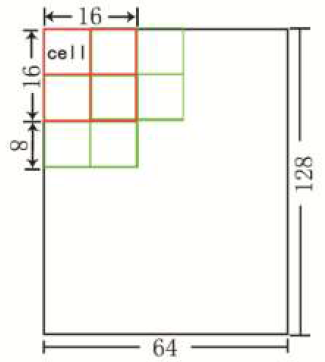## 4.2 灰度共生矩阵

p(i,j,d,θ)=[(x,y),(x+Dx,y+Dx)|f(x,y)=i,f(x+Dx,y+Dy)=j] p ( i , j , d , θ ) = [ ( x , y ) , ( x + D x , y + D x ) | f ( x , y ) = i , f ( x + D x , y + D y ) = j ]
<script type="math/tex; mode=display" id="MathJax-Element-93">p(i,j,d,θ)={[(x,y),(x+Dx,y+Dx) | f(x,y)=i,f(x+Dx,y+Dy)=j]}</script>式中： x,y=0,1,2,,N1 x , y = 0 , 1 , 2 , … , N − 1 <script type="math/tex" id="MathJax-Element-94">x,y=0,1,2,…,N-1</script>是图像中的像素坐标； i,j=0,1,2,,L1 i , j = 0 , 1 , 2 , … , L − 1 <script type="math/tex" id="MathJax-Element-95">i,j=0,1,2,…,L-1</script>是灰度级； DxDy D x ， D y <script type="math/tex" id="MathJax-Element-96">Dx，Dy</script>是位置偏移量；d为生成灰度共生矩阵的步长；θ生成方向，可以取 0,45,90,135 0 ° , 45 ° , 90 ° , 135 ° <script type="math/tex" id="MathJax-Element-97">0^°,45^°,90^°,135^°</script>四个方向，从而生成不同方向的共生矩阵。要使其特征值不受区域范围的影响，还需对此灰度共生矩阵进行归一化处理：
ASM=i=0L1j=0L1[p(i,j,d,θ)] A S M = ∑ i = 0 L − 1 ∑ j = 0 L − 1 [ p ( i , j , d , θ ) ]
<script type="math/tex; mode=display" id="MathJax-Element-98">ASM=\sum_{i=0}^{L-1}\sum_{j=0}^{L-1}[p(i,j,d,\theta )]</script>
ENT=i=0L1j=0L1p(i,j,d,θ)Inp(i,j,d,θ) E N T = ∑ i = 0 L − 1 ∑ j = 0 L − 1 p ( i , j , d , θ ) I n p ( i , j , d , θ )
<script type="math/tex; mode=display" id="MathJax-Element-99">ENT=\sum_{i=0}^{L-1}\sum_{j=0}^{L-1}p(i,j,d,\theta )Inp(i,j,d, \theta)</script>
ENT=i=0L1j=0L1(ij)2[p(i,j,d,θ)] E N T = ∑ i = 0 L − 1 ∑ j = 0 L − 1 ( i − j ) 2 [ p ( i , j , d , θ ) ]
<script type="math/tex; mode=display" id="MathJax-Element-100">ENT=\sum_{i=0}^{L-1}\sum_{j=0}^{L-1}(i-j)^2 [p(i,j,d,\theta )] </script>
ASM=i=0L1j=0L1p(i,j,d,θ)[1+(ij)2] A S M = ∑ i = 0 L − 1 ∑ j = 0 L − 1 p ( i , j , d , θ ) [ 1 + ( i − j ) 2 ]
<script type="math/tex; mode=display" id="MathJax-Element-101">ASM=\sum_{i=0}^{L-1}\sum_{j=0}^{L-1}\frac{p(i,j,d,\theta )}{[1+(i-j)^2]}</script>由灰度共生矩阵能够导出许多纹理特征，可以计算出14种灰度共生矩阵特征统计量。对图像上的每一像元求出某种邻域的灰度共生矩阵，再由该灰度共生矩阵求出各统计量．就得到对应纹理图像的统计量。若干统计量可以组成图像分类的特征向量。

# 6. 分类结果评价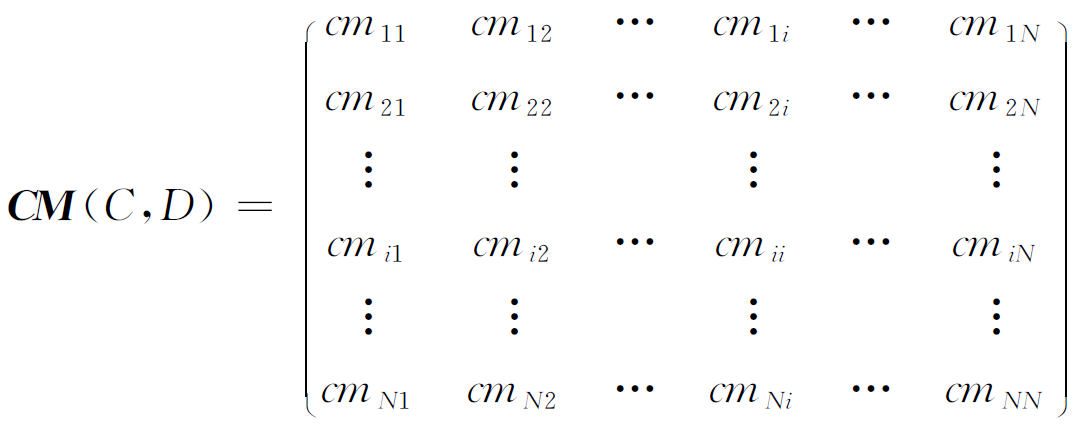(Acc)=×100% 准 确 率 ( A c c ) = 正 确 分 类 的 样 本 数 量 测 试 样 本 的 总 数 × 100 %
<script type="math/tex; mode=display" id="MathJax-Element-56">准确率(Acc)=\frac{正确分类的样本数量}{测试样本的总数}×100\%</script>

06-052万+03-241641
05-094566
12-272863
05-161万+
07-22
05-04
©️2020 CSDN 皮肤主题: Age of Ai 设计师:meimeiellie